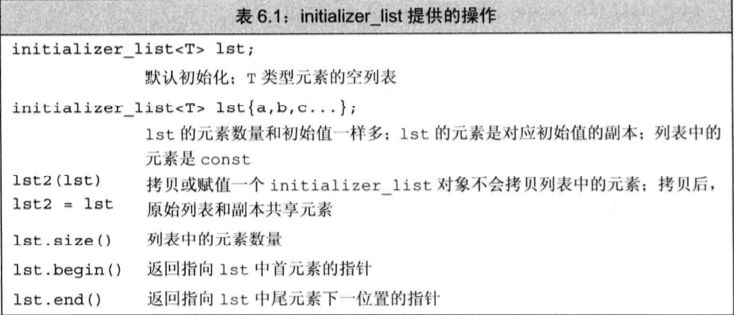# STL 库

STL库笔记。包括`iostrem` `string` `vector`

## iostream

`<iostream>`中常用的对象有`cin` `cout` `cerr` `clog`，系统通常将程序运行的控制台与这些对象关联起来。因此，从`cin`中读取数据，即是从程序正在运行的控制台读取；往`cout` `cerr` `clog`写入数据时，即是往控制台写入数据。控制台会将写入的数据显示出来。

`<iostream>`的对象实现都自带了一个缓冲区`buffer`，比如通过`cout`输出到控制台的数据都会在`buffer`中停留，等待`buffer`区满了再一次性显示到控制台。在调试时添加打印语句，应该立即刷新 `buffer`，输出内容。否则，如果程序奔溃，打印语句可能还留在`buffer`中，从而导致关于程序崩溃位置的错误推断。`cout << endl;`结束当前行，并将`buffer`中的内容强制输出到控制台。

`<<`左侧必须是`<ostream>`对象，右侧是要打印的值。`<<`运算后仍然返回这个`<ostream>`对象，所以可以连续调用，比如`cout << v1 << v2;`

`>>`左侧必须是`<istream>`对象，右侧为一变量，它从控制台中读取数据，并存入右侧对象中。`>>`运算后仍然返回这个`<istream>`对象。

``````string word;

// 反复读取单词，直至文件结尾
while( cin >> word )
cout << word << endl;

// 反复读取一行，直至文件结尾
string line;
while( getline(cin,line) ) // getline会读取换行符，但不会将换行符存入到string对象中
{
if( !line.empty() ) // 输出非空行
cout << line << endl;
}``````

`istream`的读取模式是，读取用户输入，直到遇到第一个空白字符（空格，换行符，制表符），作为一次读取。多个空白字符连续的情况，只视作一个空白字符。

## string

• C风格字符串就是一个以 `\0` 字符结尾的字符数组

• `string` 支持完全随机访问

• `string` 隐藏了字符串的数组性质，能够像处理普通变量那样处理字符串

• `string` 可以赋值 字符数组不能

``````char dog = {'b','e','a','u','x',' ','i','I'};  // not a string
char cat = {'f','a','t','a','s','s','a','\0'}; // a string
char bird = "codekissyoung"; // the \0 is understood
char fish[] = "Bubbles"; // let the compiler count
char shirt_size = 'S'; // a char
char shirt_size = "s"; // error !!!

string name = "codekissyoung";
printf("name : %s\n", name.c_str()); // string 类型 转换成 c 字符串
s.substr(i,j); 截取 [i,j)范围内的字符串
getline(is,s); 从 is 中读入一行，存储在 s 中

string str1; // 初始化字符串为空的变量
string str2 = "codekissyoung";
string str3;
str1 = str2; // 赋值
str3 = str1 + str2; // str1 拼接 str2 后，再赋值给 str3
str1 += str2; // 将str2添加到str1后面
int len1 = str1.size(); // 获取字符串长度
string adc( 100, '*' ); // 初始化字符串为 100 个 * 号
string::size_type cols = abc.size() + 2; // 保存字符串的长度``````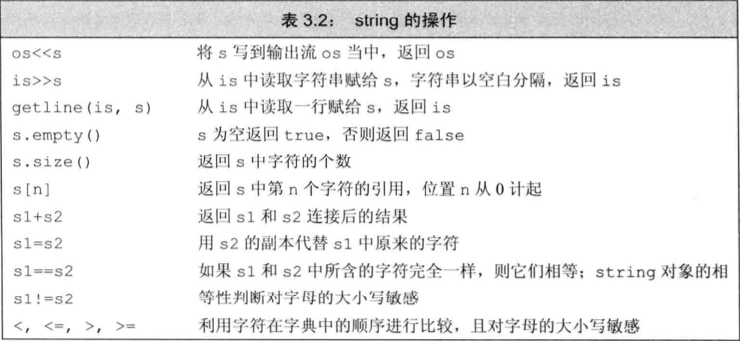`cin >> str;``string`对象会自动忽略开头的空白字符，并从第一个真正的字符开始读起，直到遇见下一处空白为止。

`str.size()`返回的类型`string::size_type`类型，很明显是`unsigned`类型，假如有一`int`负值 `n`，那么`str.size() < n`的判定可能为`true`,因为`signed`类型与`unsigned`类型进行比较，`signed`类型会转换成`unsigned`类型，即一个较大的正数。

## vector

`vector`不仅存储数据元素，还存储元素的个数。第一个数据元素的索引号是`0`、第二个是`1`,依次类推。所有的数据元素要求为同一种数据类型。

``````vector<数据类型> 变量名( 元素个数 );
vector<double> vec;
vec.push_back( 4.5 );   // 添加新元素到`vector`中

// 遍历vector中元素
for(int i = 0; i < vec.size(); ++i)
cout << vec[i] << endl;``````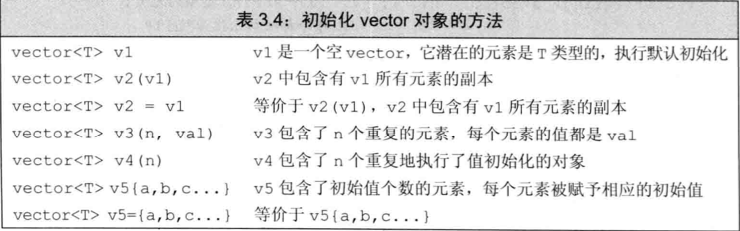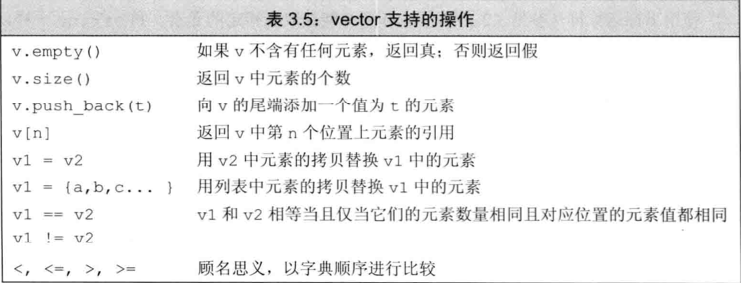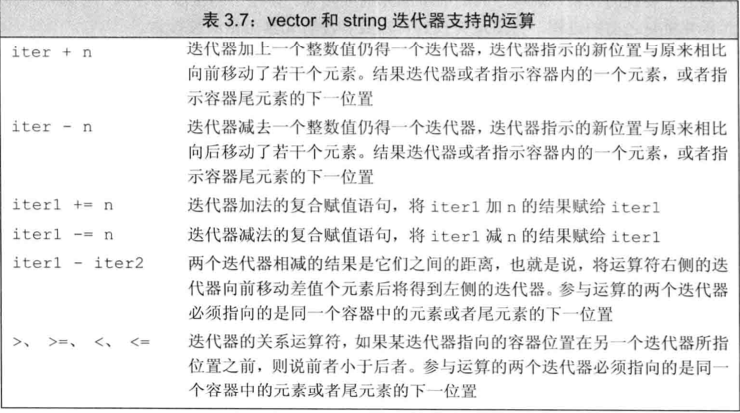## vector、list、string

``````container<T>::iterator
container<T>::const_iterator
container<T>::size_type 用来保存这个容器可能存在的最大实例长度
c.begin() 返回指向容器第一个元素的迭代器
c.end()
c.rbegin()
c.rend()
container<T> c; 声明一个容器
container<T> c(c2); 使用 c2 声明一个容器
container<T> c(n); 声明一个有 n 个元素的容器
container<T> c(n,t); 声明一个有 n 个元素的容器，每个元素为 t
container<T> c(b,e);
c = c2; 两个容器之间赋值
c.size(); 容器内元素个数
c.empty(); 用于判定 c 中是否含有元素
c.insert(d,b,e); 复制位于[b,e)区间的元素，插入到 容器 c 中 d 之前的位置
c.erase(it); 删除迭代器指向的元素，并且返回指向后一个元素的迭代器
c.erase(b,e); 删除某个区间[b,e)指向的元素
对于<list>来说，指向被删元素的迭代器会失效
对于`vector`和string来说，被删元素后面的迭代器都会失效
c.push_back(t); 在 c 末尾添加一个元素，这个元素就是 t

c[n]; 从容器 c 中取出位于位置 n 的字符串

*it 引用迭代器指向的位置处的值
it->x 访问迭代器指向的对象的成员
++it;
it++; 迭代器自增 1 ,指向容器中的下一个元素
b == c;
b != c; 判断`vector`是否相等

v.reverse(n); 保留空间以保存 n 个元素
v.resize(n);

list 类型是为了高效地在容器中任何位置插入和删除元素而被优化的
l.sort();
l.sort(cmp); 使用 cmp 来排序 list 中的元素``````

## cctype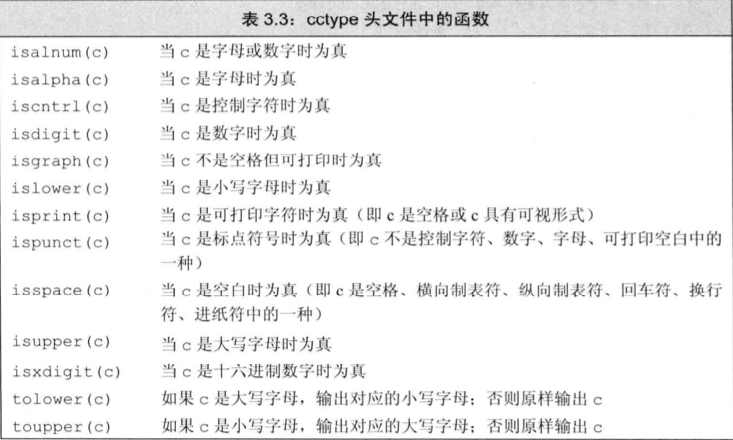## stdexcept 异常类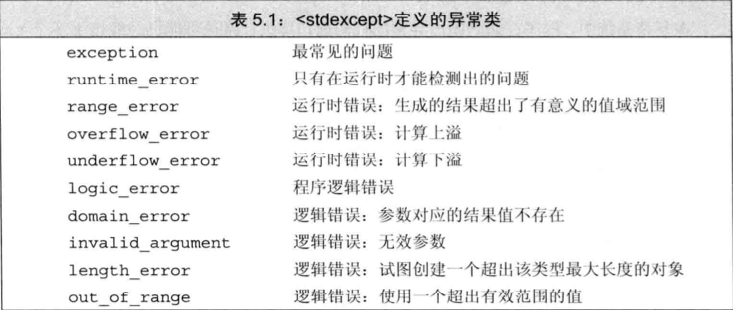## initializer_list 用于参数个数不确定的情况

``````// 初始化列表用于传值
typedef unsigned int ErrCode;
void error_msg( ErrCode e, std::initializer_list<std::string> il )
{
std::cout << e << ": ";
for(const auto &elem : il )
std::cout << elem << " ";
std::cout << std::endl;
}
error_msg( ErrCode(42), {"function xxx", "okay", "xxxxxxx"});

// 初始化列表用于函数返回
vector<string> process()
{
if( expected.empty() )
return {};
else if( expected == actual )
return {"functionX" , "okay"};
else
return {"functionX", expected, actual};
}``````